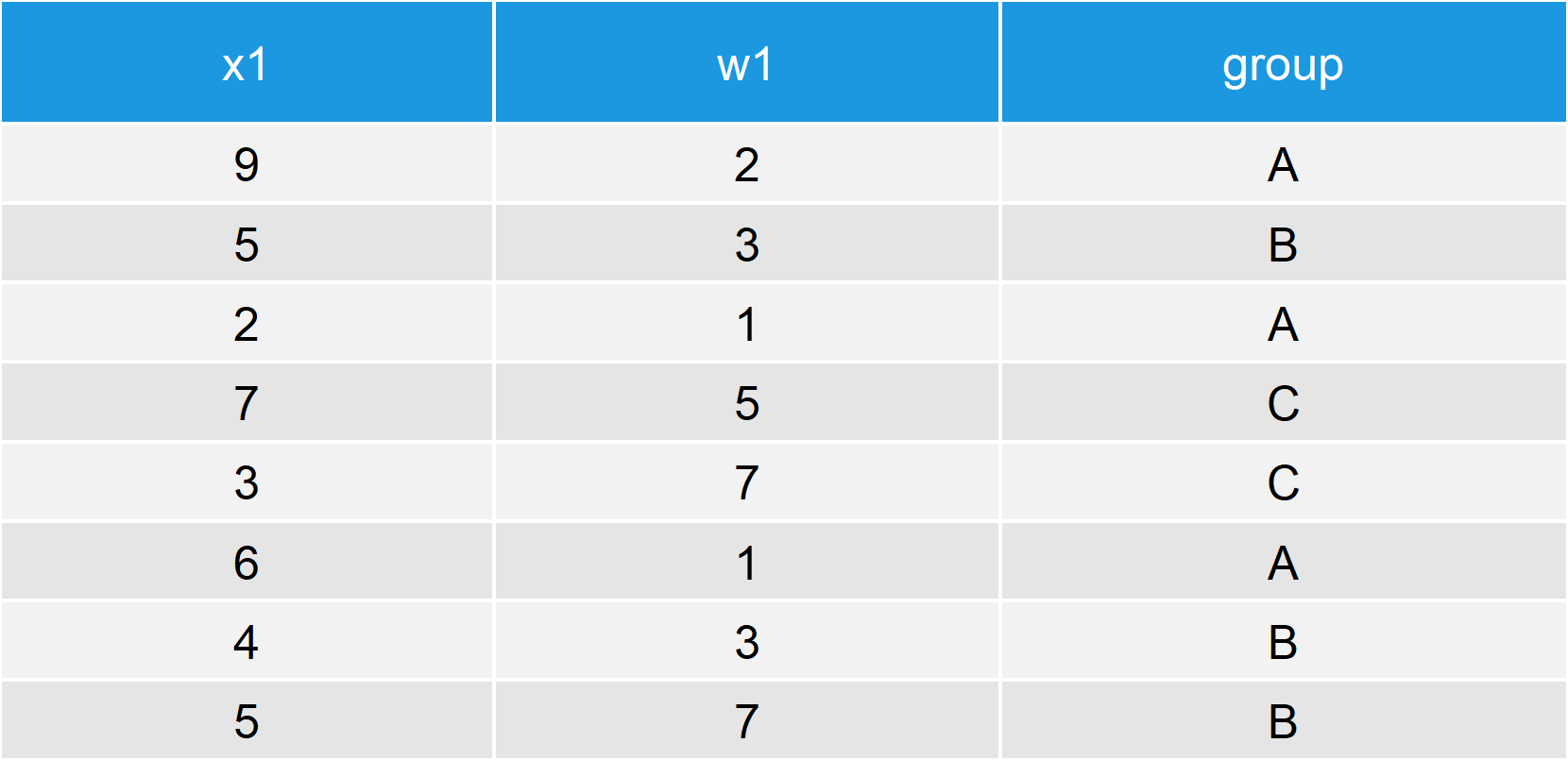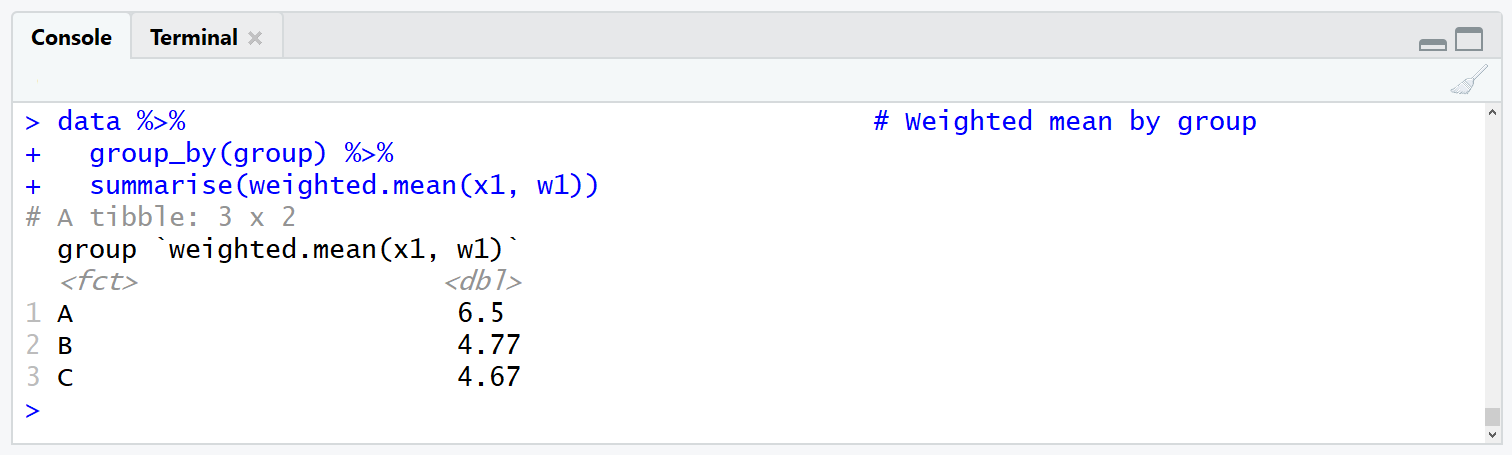# Weighted Mean in R (5 Examples)

This tutorial explains how to compute the weighted mean in the R programming language.

The tutorial is mainly based on the weighted.mean() function. So let’s have a look at the basic R syntax and the definition of the weighted.mean function first:

Basic R Syntax of weighted.mean():

`weighted.mean(x, weights)`

Definition of weighted.mean():

The weighted.mean function computes the weighted arithmetic mean of a numeric input vector.

You are here for the answer, so let’s move on to the examples!

## Example 1: Basic Application of weighted.mean Function in R

First, we need to create some example data and a vector with corresponding weights. Consider the following example data:

```x1 <- c(9, 5, 2, 7, 3, 6, 4, 5)                    # Create example data
w1 <- c(2, 3, 1, 5, 7, 1, 3, 7)                    # Create example weights```

Now, we can use the weighted.mean command to compute the weighted mean of this data:

```weighted.mean(x1, w1)                              # Apply weighted.mean function
# 4.965517```

As you can see based on the RStudio console output, the weighted mean of our data is 4.965517.

## Example 2: Handle NAs with weighted.mean Function

A typical problem is the occurrence of missing values in our data (i.e. NA values). Let’s extent our example data with NA values to simulate this situation:

```x2 <- c(x1, NA)                                    # Create vector with NA
w2 <- c(w1, 3)                                     # Extend weights vector```

If we now apply the weighted.mean function as in Example 1, the RStudio console returns NA:

```weighted.mean(x2, w2)                              # weighted.mean with NA
# NA```

Fortunately, we can easily ignore NA values in our data with the na.rm option of the weighted.mean function:

```weighted.mean(x2, w2, na.rm = TRUE)                # Remove missing values
# 4.965517```

## Example 3: Compute Weighted Means by Group

Often we are only interested to know the weighted mean for a subgroup of our data. Consider the following example data:

```group <- c("A", "B", "A", "C", "C", "A", "B", "B") # Create group indicator
data <- data.frame(x1, w1, group)                  # Create data frame```Table 1: Example Data with Numeric Column, Weights & Group Indicator.

To compute the weighted mean by group we can use the functions of the dplyr package. Let’s install and load the package to R:

```install.packages("dplyr")                          # Install dplyr package

Now, we can calculate the weighted mean with the following R code:

```data %>%                                           # Weighted mean by group
group_by(group) %>%
summarise(weighted.mean(x1, w1))```Figure 1: dplyr Tibble Containing Weighted Means.

As you can see based on Figure 1, the previous R code returns a tibble with the weighted means by group to the RStudio console.

## Alternative 1: weightedMean Function of matrixStats Package

The beauty of R is that there are always several ways to achieve a goal. Of cause, this is also true for the computation of the weighted mean. In the following two examples, I will therefore show some alternatives to the weighted.mean function.

Let’s start with the weightedMean function of the matrixStats package. First, we need to install and load the package…

```install.packages("matrixStats")                    # Install matrixStats package

…and then we can apply the weightedMean R function as follows:

```weightedMean(x1, w1)                               # Apply weightedMean function
# 4.965517```

The same result as in Example 1 – looks good!

## Alternative 2: wt.mean Function of SDMTools Package

Another alternative is the wt.mean function of the SDMTools package:

```install.packages("SDMTools")                       # Install SDMTools package

After installing and loading the R package, we can apply the wt.mean command as follows:

```wt.mean(x1, w1)                                    # Apply wt.mean function
# 4.965517```

Again, the same result.

## Video, Further Resources & Summary

Still have problems on this topic? Check out the video below where I explain the steps of this article more detailed:

Please accept YouTube cookies to play this video. By accepting you will be accessing content from YouTube, a service provided by an external third party.If you accept this notice, your choice will be saved and the page will refresh.

This article illustrated how to compute weighted means in the R programming language. In case you want to learn more the theoretical research concept of the weighted arithmetic mean, I can recommend the following video tutorial of the MySecretMathTutor YouTube channel:

Please accept YouTube cookies to play this video. By accepting you will be accessing content from YouTube, a service provided by an external third party.If you accept this notice, your choice will be saved and the page will refresh.

In addition, you may also have a look at some of the related R tutorials that I have published on this website:

I hope this tutorial contained the content you were looking for. However, don’t hesitate to let me know in case you have any comments or questions.

Subscribe to the Statistics Globe Newsletter

•Altamash Bashir
April 7, 2020 4:55 pm

Your description for calculating wtd mean was helpful but it was only with one variable. How to find weighted standard deviation or mean for many columns in the database

•April 8, 2020 5:47 am

Hi Altamash,

I’m sure there are more efficient ways, but one solution might be to use a for-loop. Consider the following example:

```x1 <- c(9, 5, 2, 7, 3, 6, 4, 5)
x2 <- c(7, 7, 9, 5, 2, 7, 3, 6)
w1 <- c(2, 3, 1, 5, 7, 1, 3, 7)

data <- data.frame(x1, x2, w1)

weighted_columns <- numeric()
for(i in 1:(ncol(data) - 1)) {
weighted_columns[i] <- weighted.mean(data[ , i], w1)
}```

The weighted means of x1 and x2 are stored in the data object weighted_columns.

I hope that helps!

Joachim

•yoyong
April 22, 2021 10:13 pm

Hi Joachim.

If you have another column, which is gender <- c("m","f","f","m","f","f","m","f"), how do you include a grouping variable in your code?

Thanks.

•Viswanathan
May 29, 2021 2:47 am

Hi Joachim,
Can we use the aggregate() function? Because I generally use the fun for calculating the mean for a grouped datasets.

•May 31, 2021 7:46 am

Hey Viswanathan,

Thank you for the nice comment!

I assume that it should be possible to use the aggregate function, but I’m not sure how to do it. However, in case you want to calculate the weighted mean by group you may also use the code of Example 3.

Regards

Joachim

•santosh
April 26, 2023 4:31 pm

how to calculate for next 10 years forecast by using weighted.mean
As we are able to forecast for next year only

•April 27, 2023 12:21 pm

Hello Santosh,

I guess as long as you can predict the weights, you can calculate it. I don’t see such an example in the tutorial. Is it about your data? If so, please give me some more details to help you.

Regards,
Cansu

•santosh
April 28, 2023 12:31 am

the data set is like we have counts for 10 years from 2012 to 2022 and i am trying to predict for 2030 what will be the forecasted value.
Year Count
2012 6256
2013 6455
2014 6730
2015 7127
2016 7841
2017 8082
2018 8250
2019 8896
2020 8906
2021 9236
2022 9528

•April 28, 2023 7:23 am